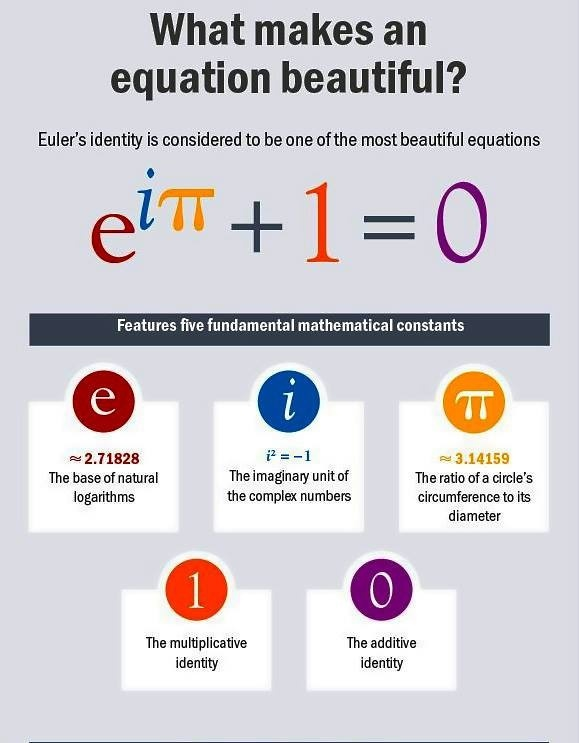# Most Beautiful Equation in Mathematicse to the pi i for dummies

Quora QA
Wikipedia### Larry Battle

I love to program, and discover new tech. Check out my stackoverflow and github accounts.

# Ratio.js – Fractions for javascriptHey Everyone,
I created a project called Ratio.js. Basically the goal of the project is to provide an simple object for dealing with fractions in javascript.
Check it out here and tell me what this.

## Ratio.js

Sample Code

``` ```  // converts decimal values into the form of a fraction. a = Ratio.parse(1/2); a.toString() == "1/2";   // converts strings in the form of "a/b" to a fraction a/b. a = Ratio.parse( "1/2" ); a.toString() == "1/2";   var result = Ratio.parse( 12.12121212121212 ).reduce().toString(); result == "400/33";``` ```

## Simple Demo### Larry Battle

I love to program, and discover new tech. Check out my stackoverflow and github accounts.

# How to convert a repeating decimal to a fractionHere are 4 simple steps to convert a repeating decimal to a fraction.

Step 1: Check to see if the number has a repeating decimal. Stop if it doesn’t and do normal conversion.

Step 2: Split the decimal into 3 parts; i, x, r. Such that the decimal equals `i.x(r)*`.

Step 3: Create a fraction in the form `a/b`.
a = ((ixr as int) – (ix as int))
b = ((10^x.length)*(10^r.length – 1))

Step 4: (optional) Reduce the fraction by dividing by the greatest common denominator.

Example:
4/3 = 1.333… which sets i = “1”, x = “”, r = “3”.
a = (13 – 1) = 12, b = ((10^0)*(10^1 – 1)) = 9
a/b = 12 / 9 = 4/3

Ratio.js does this for you when the `reduce` function is called.

Example using Ratio.js:

``` ```Ratio.parse( "1/3" ).reduce().toString() === "1/3"; Ratio.parse( 4/3 ).reduce().toString() === "4/3"; Ratio.parse( 0.123451234512345 ).reduce().toString() === "4115/33333"; Ratio.parse( 0.987987989798979897 ).reduce().toString() === "978108109901/990000000000";``` ```

## DEMO### Larry Battle

I love to program, and discover new tech. Check out my stackoverflow and github accounts.

# Code of the Day: Javascript Decimal Expansion a.k.a DivisionToday’s Code of the Day is about decimal expansion, which is just division.

So you might be asking yourself, “if decimal expansion is divsion. Then why not use a/b?”.
Well the problem is that Javascript has a ton of problems when dealing with floating point operations because of the way they are stored.

Examples:

``` ```var a = 1/3; a.toString() // returns "0.3333333333333333" a.toFixed(25); // returns "0.3333333333333333148296163" 0.1 + 0.2; // returns 0.30000000000000004``` ```

Source for decimalExpansion()

``` ```// borrowed from jQuery 1.7.2 var isNumeric = function(val){ return !isNaN(parseFloat(val)) && isFinite(val); }; /** * @author Larry Battle <http://bateru.com/news/contact-me> * @date May 16, 2012 * @license MIT and GPLv3 */ //decimalExpansion returns a string representation of a divided by b to a fixed length. // All the paramaters must be whole numbers. // Example: decimalExpansion( 1, 3, 3 ) === "0.333" var decimalExpansion = function (top, bottom, decLength) { if (!isNumeric(top) || !isNumeric(bottom) || !isNumeric(decLength) || !bottom) { return null; } var sign = ((top * bottom) != Math.abs(top * bottom)) ? "-" : ""; top = Math.abs(top); bottom = Math.abs(bottom); decLength = Math.abs(decLength);   var result = Math.floor(top / bottom), remainder = top % bottom, maxDecimal = 100, i = Math.min(Math.max(0, decLength), maxDecimal) + 1;   if (1 < i) { result += "."; while (i--) { top = remainder * 10; remainder = top % bottom; result += "" + Math.floor(top / bottom); } result = result.replace(/(\d)(\d)\$/, function (match, a, b) { return +b > 4 ? +a + 1 : a; }); } return sign + result; };``` ```

Test cases:

Demo:

Here are excellent links over the topic.
Wolfram MathWorld: Decimal Expansion
Wikipedia.org: Fraction (mathematics)
Oracle: What Every Computer Scientist Should Know About Floating-Point Arithmetic### Larry Battle

I love to program, and discover new tech. Check out my stackoverflow and github accounts.